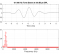## Matlab - How to plot magnitude errorStarted by 7 years ago8 replieslatest reply 7 years ago457 views

I'm trying to plot the magnitude (and phase) response error between an analog filter and digital filter.

I have this code:

%pkg load signal

fs = 44100;

%% analog model
a2 = [0.000015 1];
b2 = [0.000050 1];
Ds = tf(a2, b2);
Ds = Ds/dcgain(Ds);

%% digial 1
B1 = [1 -0.62786881719628800 0];
A1 = [-0.08782333709141920 0.45995451989513100 0];
Dz = tf(A1, B1, 1/fs);
Dz = Dz/dcgain(Dz);

%% digital 2
B2 = [1 0.8868033819284362 -0.312597640666886 -0.3462305068062579 -0.04427163895789011];
A2 = [0.4601686131709282 0.6087942052682088 0.1563216019782706 -0.03386380454131521 -0.007717020378690522];
Dz2 = tf(A2, B2, 1/fs);
Dz2 = Dz2/dcgain(Dz2);

%% plot
wmin = 2 * pi * 20; % 20Hz
wmax = 2 * pi * (fs/2);

figure(1);
bode(Ds, 'r', Dz, 'm', Dz2, 'g', {wmin, wmax});
legend('analog', 'Dz', 'Dz2','location', 'northwest');
grid on;

% plot errors
e1 = Dz/Dz2;
figure(2);
bode(e1, 'r', {wmin, wmax});
legend('Dz/Dz2','location', 'northwest');
grid on;

in #Octave (#Matlab) and I would not like to use freqz()/freqs() for the task.

Dz/Dz2 seem to give some close result for magnitude difference between these two in calculation but, is this method suitable for to calculate magnitude 'error' between two filters?Large image - https://postimg.org/image/cy3v9wi75/

Two first plots represents the responses above 1e+4, two last plots represents the difference between Dz and Dz2.

Problem is that calculation Ds/Dz can't be done (error: lti_group: systems must have identical sampling times) so I have no information if the result is usable (other but comparing the plots manually). If sampling time info is added for the Ds then the result is wrong.

So, I suppose, to get the results I'm after, Ds should be 'transformed' to same type data as what Dz's are ? How is that done without loosing responses of the analog filter?

[ - ]Rather than try to compute e=F/G as a transfer function (F/G = Dz/Dz2 in your case), why not evaluate them at specific test frequencies? In other words evaluate Fw = F(w) and Gw = G(w) and then plot mag_error_dB = 20*log10(abs(Fw/Gw)).

Otherwise you can run into problems trying to create viable transfer functions. (as you discovered, trying to take quotients of Ds/Dz where s is continuous and z is not)

[ - ]I don't have much knowledge in DSP, related math nor using Octave so, could you open a bit the evaluation of Fw = F(w) and Gw = G(w). Maybe some little example?

[ - ]I'm not familiar with either Matlab or Octave (I use Scilab), but basically you want to find the calls that let you evaluate the transfer functions at specified frequencies.  Then you want to get a vector of frequency responses for each, using the same vector of frequencies.  Then divide the frequency responses point by point and plot.

[ - ]Why do you not want to use freqz/freqs for the task?  If they do what I think they do (calculate the frequency response point-by-point) that's probably the best result you're going to get.

Note that you're comparing apples to oranges -- the frequency response of a digital filter inside the digital domain is not the same as the frequency response of a sampled-time system with that digital filter embedded therein -- you need to account for the anti-aliasing filter, the effect of the DAC's ZOH, and any reconstruction filtering.

If you wanted, say, to compare the frequency response of a certain analog filter on a board vs. a certain digital filter on a bit of DSP hardware on a board with ADC, DAC, and associated signal conditioning electronics, then unless your sampling rate is way high compared to your cutoff frequencies you'd have to take that associated signal conditioning electronics into account to get a really accurate picture.

[ - ]As mentioned above I'm not familiar with Octave/Matlab.

I tried to find some freqz/freqs based solutions (example code) matching with this subject but, all those I found used [H, w] = freqs(...) which is not supported by Octave and I couldn't get the found codes working other way in Octave.

ATM, I use 24th order filters (generated from analog model using MIM and PIM methods (my other thread here) which both I finally got working (these generated filters mathes the magnitude (MIM) and phase (PIM) excellently in range 20Hz-20kHz)) for to simulate the analog model and then divide as mentioned in my 1st post.

BTW, it's digital domain I'm dealing with ... just trying to prepare few digital IIR filters which (magnitude response) closely matches the digital 'analog model' of it (matcing both, magnitude and phase simultaneously looks very hard job to do).

[ - ]If you're working with audio, particularly stored audio, you may find it helpful to try matching the gain and phase of a slightly delayed version of your analog filter.  Or not -- I'm just tossing this out for you to play with if you wish.

[ - ]I am more familiar with Matlab than Octave.  However, it does look like Octave supports the "freqz" function, which appears to be what you want based on your last paragraph.  See Octave - FREQZ.

As jms_nh indicated, you could compare the magnitude and phase responses of several digital IIR filters by computing the response for a set of frequencies.  The "freqz" function readily supports computing these responses for a given filter, defined by the "b" and "a" coefficients, by giving the required values in the "w" vector argument.  "w" values can be given in radians per sample or Hertz.

The syntax would be:

"[h, w] = freqz(b, a, w)" for a set of normalized frequencies.

or

"[h, w] = freqz(b, a, f, Fs)" for a set of frequencies in Hertz.

You could then plot the magnitude and phase responses for the IIR digital filters you wanted to compare using syntax similar to:

"figure; hold on; stem(w1, 20*log20(abs(h1))); stem(w2, 20*log20(abs(h2)), 'r');"

for a set of normalized frequencies.

[ - ]